# Divide using long division. 8x3 - 38x + 3 2x - 5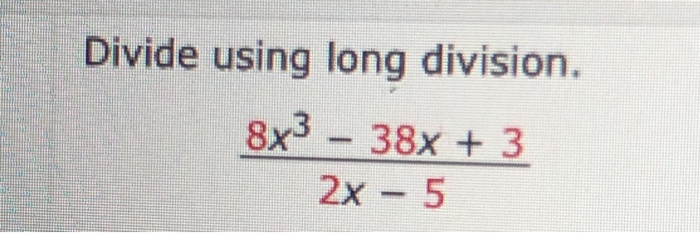Divide using long division. 8x3 - 38x + 3 2x - 5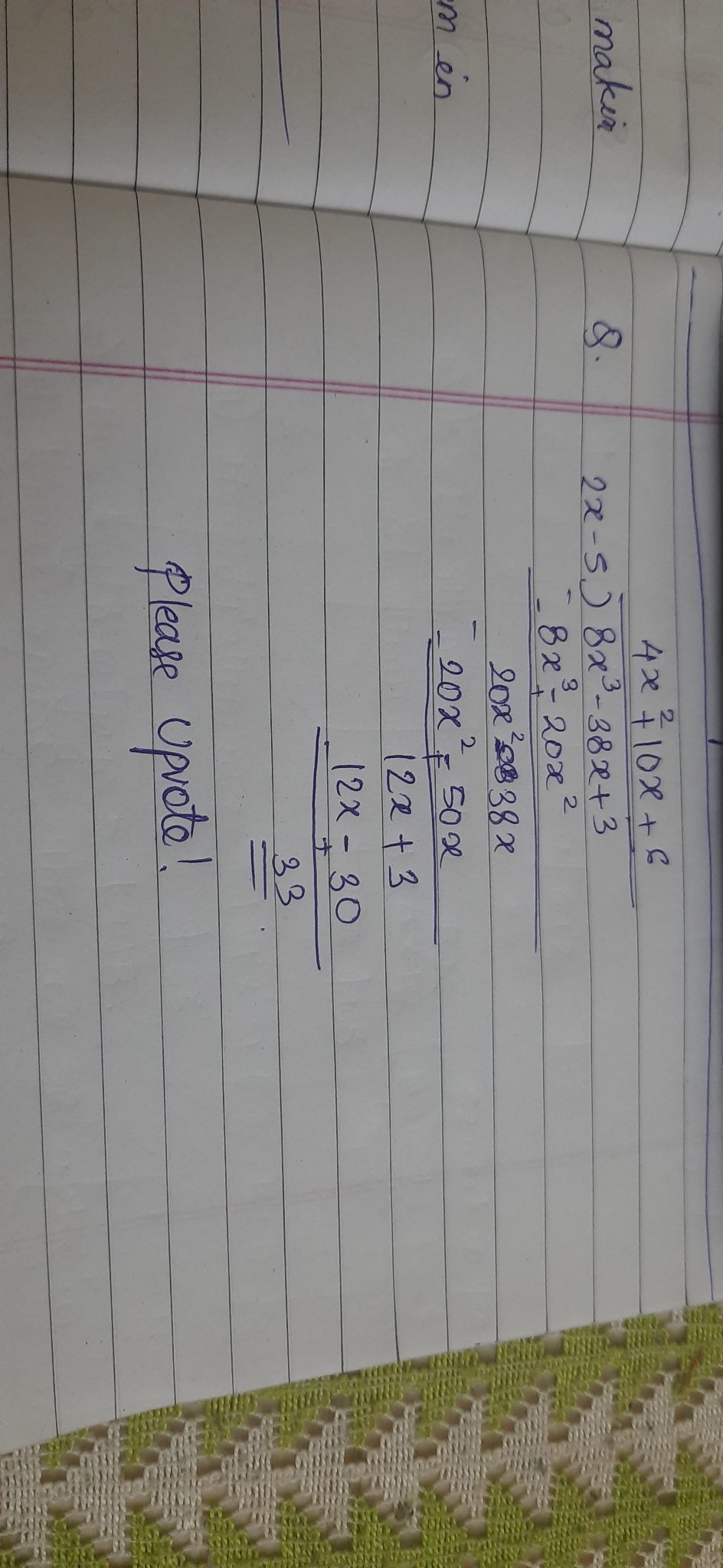##### Add Answer of: Divide using long division. 8x3 - 38x + 3 2x - 5
Similar Homework Help Questions
• ### Divide using long division

divide using long division and clearly identify the quotient and remainderx3 +2x-4/x2 -x

• ### Divide (2x^3-8x^2-4x-6)/(x-3) using synthetic division

Divide (2x^3-8x^2-4x-6)/(x-3) using synthetic division

• ### Use synthetic division to divide

3. Use synthetic division to divide 8x3 -20x3 - 14x2 + 8x + 1 by x + 1.a. 8x3 + 28x2 - 14x R11b. 8x3 - 28x2 + 14x - 6 R7c. 8x3 + 36x2+ 18x + 10 R9d. 8x3 + 28x2 - 14x + 8

• ### divide by long division

divide by long division.divide x^3 + 4x^2 - 3x - 12 by x^2 - 3

• ### long division

Use long division to divide 3x3 – 12x2 + 2x – 5 by (x – 4).

• ### Divide z° +61 by Z+ 4 using Long Division and check.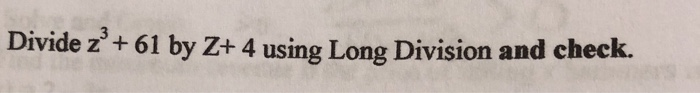Divide z° +61 by Z+ 4 using Long Division and check.

• ### 10) Divide zº + 61 by Z+ 4 using Long Division and check.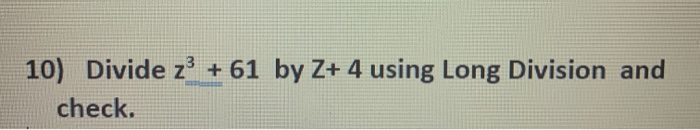10) Divide zº + 61 by Z+ 4 using Long Division and check.

• ### Solve for x using factor theorem and long division.   -2x^3-12^2+2x+60=0

Solve for x using factor theorem and long division.   -2x^3-12^2+2x+60=0

• ### ORATIONAL EXPRESSIO Polynomial long division: Problem type 2 Divide. (8x' + 24x² + 14x-1) + (2x+3)...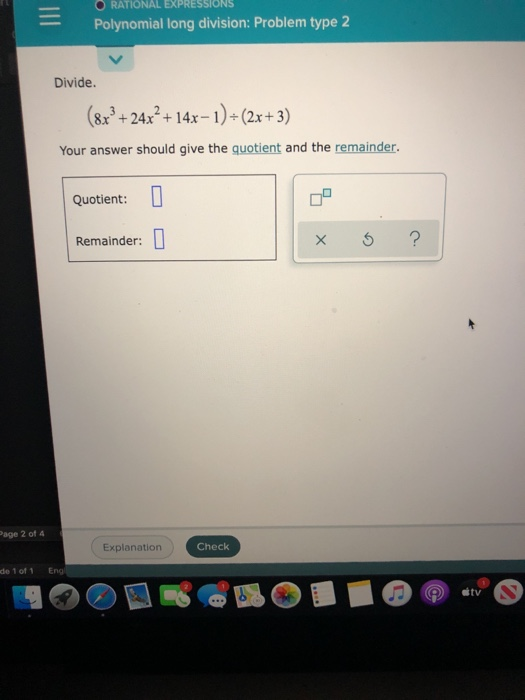ORATIONAL EXPRESSIO Polynomial long division: Problem type 2 Divide. (8x' + 24x² + 14x-1) + (2x+3) Your answer should give the quotient and the remainder. Quotient: Remainder: 0 ? Page 2 of 4 Explanation Check de 1 of 1 Eng tv

• ### When long division is used to divide 16x3 - 3x + 5 by 4x + 3,...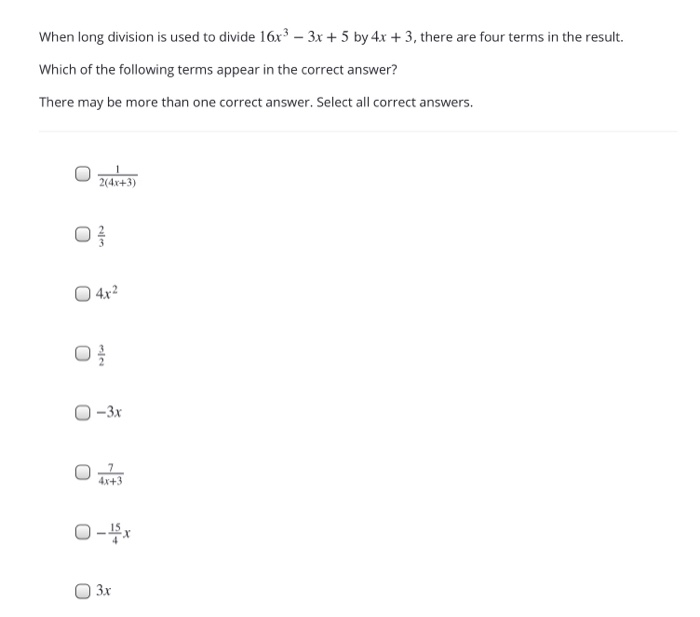When long division is used to divide 16x3 - 3x + 5 by 4x + 3, there are four terms in the result. Which of the following terms appear in the correct answer? There may be more than one correct answer. Select all correct answers. 2(4x+3) O 04x2 0-3x O-X 3x

Free Homework App